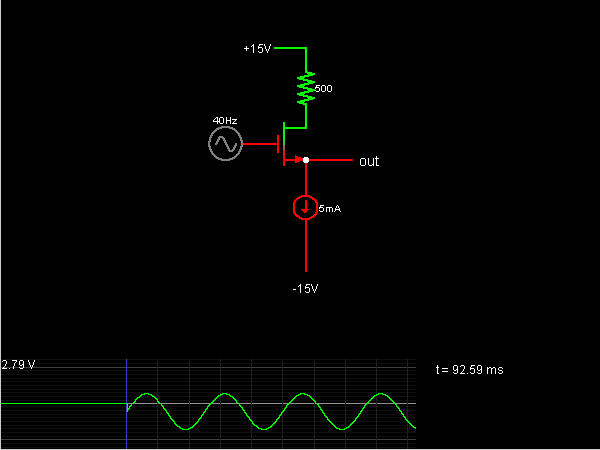# Circuit Simulator - Source Follower

Source FollowerCircuit Description:
This is a source follower or buffer amplifier circuit using a MOSFET. The output is simply equal to the input minus about 2.2V. The advantage of this circuit is that the MOSFET can provide current and power gain; the MOSFET draws no current from the input. It provides low output impedance to any circuit using the output of the follower, meaning that the output will not drop under load. Its output impedance is not as low as that of an emitter follower using a bipolar transistor (as you can verify by connecting a resistor from the output to -15V), but it has the advantage that the input impedance is infinite.

The MOSFET is in saturation, so the current across it is determined by the gate-source voltage. Since a current source keeps the current constant, the gate-source voltage is also constant.

Discussion:
Be the first person to comment on this question !